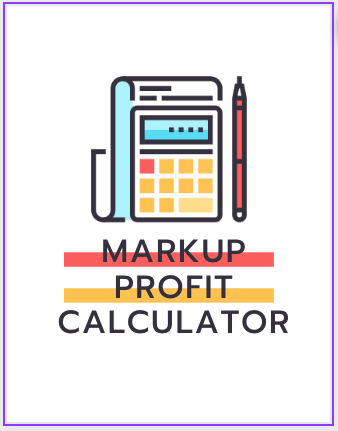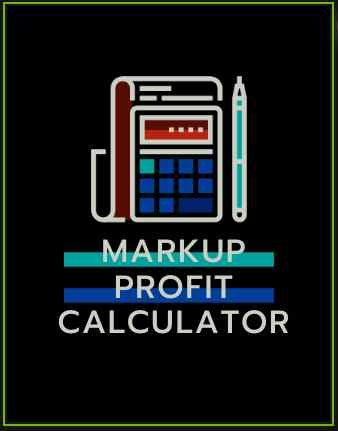# The GST Markup and Profit Calculator

## What does this Calculator do?

This is a very useful Calculator or tool, to help retailers and store owners set the price of their goods.

Very often, it is easer and more user friendly selling a product when it is priced as a Round figure.
But this is easier said than done. Why?
The main reason is GST.

### An example

Let’s take a real example:

If your item cost you 3500, adding a 25% profit, and then adding 5% gst gives you a Selling price of Rs 4593.75.
But, It would be better to set a Markup Retail Printed of  4600.

This creates another problem. Now, You will need to calculate the Sale Price on which a 5% GST  added comes to 4600.

### The Solution

This Calculator solves this problem by allowing you to enter a Final Printed Retail price , or Markup price, and Calculate the Sale Price and corresponding GST.

In this case the Sale price before GST is calculated as 4380.95, on which a 5% gst of 219.05 brings the total Final price to 4600.

Not only that, it also calculates you Profit, both as amount and as various percentages, based on the Markup Price.Click to use

### Click the icon below to use the CalculatorArticle Contents

Index